» Code examples / Timeseries / Timeseries forecasting for weather prediction

# Timeseries forecasting for weather prediction

Authors: Prabhanshu Attri, Yashika Sharma, Kristi Takach, Falak Shah
Date created: 2020/06/23
Description: This notebook demonstrates how to do timeseries forecasting using a LSTM model.View in ColabGitHub source

## Setup

This example requires TensorFlow 2.3 or higher.

``````import pandas as pd
import matplotlib.pyplot as plt
import tensorflow as tf
from tensorflow import keras
``````

## Climate Data Time-Series

We will be using Jena Climate dataset recorded by the Max Planck Institute for Biogeochemistry. The dataset consists of 14 features such as temperature, pressure, humidity etc, recorded once per 10 minutes.

Location: Weather Station, Max Planck Institute for Biogeochemistry in Jena, Germany

Time-frame Considered: Jan 10, 2009 - December 31, 2016

The table below shows the column names, their value formats, and their description.

Index Features Format Description
1 Date Time 01.01.2009 00:10:00 Date-time reference
2 p (mbar) 996.52 The pascal SI derived unit of pressure used to quantify internal pressure. Meteorological reports typically state atmospheric pressure in millibars.
3 T (degC) -8.02 Temperature in Celsius
4 Tpot (K) 265.4 Temperature in Kelvin
5 Tdew (degC) -8.9 Temperature in Celsius relative to humidity. Dew Point is a measure of the absolute amount of water in the air, the DP is the temperature at which the air cannot hold all the moisture in it and water condenses.
6 rh (%) 93.3 Relative Humidity is a measure of how saturated the air is with water vapor, the %RH determines the amount of water contained within collection objects.
7 VPmax (mbar) 3.33 Saturation vapor pressure
8 VPact (mbar) 3.11 Vapor pressure
9 VPdef (mbar) 0.22 Vapor pressure deficit
10 sh (g/kg) 1.94 Specific humidity
11 H2OC (mmol/mol) 3.12 Water vapor concentration
12 rho (g/m ** 3) 1307.75 Airtight
13 wv (m/s) 1.03 Wind speed
14 max. wv (m/s) 1.75 Maximum wind speed
15 wd (deg) 152.3 Wind direction in degrees
``````from zipfile import ZipFile
import os

zip_path = keras.utils.get_file(origin=uri, fname="jena_climate_2009_2016.csv.zip")
zip_file = ZipFile(zip_path)
zip_file.extractall()
csv_path = "jena_climate_2009_2016.csv"

``````

## Raw Data Visualization

To give us a sense of the data we are working with, each feature has been plotted below. This shows the distinct pattern of each feature over the time period from 2009 to 2016. It also shows where anomalies are present, which will be addressed during normalization.

``````titles = [
"Pressure",
"Temperature",
"Temperature in Kelvin",
"Temperature (dew point)",
"Relative Humidity",
"Saturation vapor pressure",
"Vapor pressure",
"Vapor pressure deficit",
"Specific humidity",
"Water vapor concentration",
"Airtight",
"Wind speed",
"Maximum wind speed",
"Wind direction in degrees",
]

feature_keys = [
"p (mbar)",
"T (degC)",
"Tpot (K)",
"Tdew (degC)",
"rh (%)",
"VPmax (mbar)",
"VPact (mbar)",
"VPdef (mbar)",
"sh (g/kg)",
"H2OC (mmol/mol)",
"rho (g/m**3)",
"wv (m/s)",
"max. wv (m/s)",
"wd (deg)",
]

colors = [
"blue",
"orange",
"green",
"red",
"purple",
"brown",
"pink",
"gray",
"olive",
"cyan",
]

date_time_key = "Date Time"

def show_raw_visualization(data):
time_data = data[date_time_key]
fig, axes = plt.subplots(
nrows=7, ncols=2, figsize=(15, 20), dpi=80, facecolor="w", edgecolor="k"
)
for i in range(len(feature_keys)):
key = feature_keys[i]
c = colors[i % (len(colors))]
t_data = data[key]
t_data.index = time_data
ax = t_data.plot(
ax=axes[i // 2, i % 2],
color=c,
title="{} - {}".format(titles[i], key),
rot=25,
)
ax.legend([titles[i]])
plt.tight_layout()

show_raw_visualization(df)
``````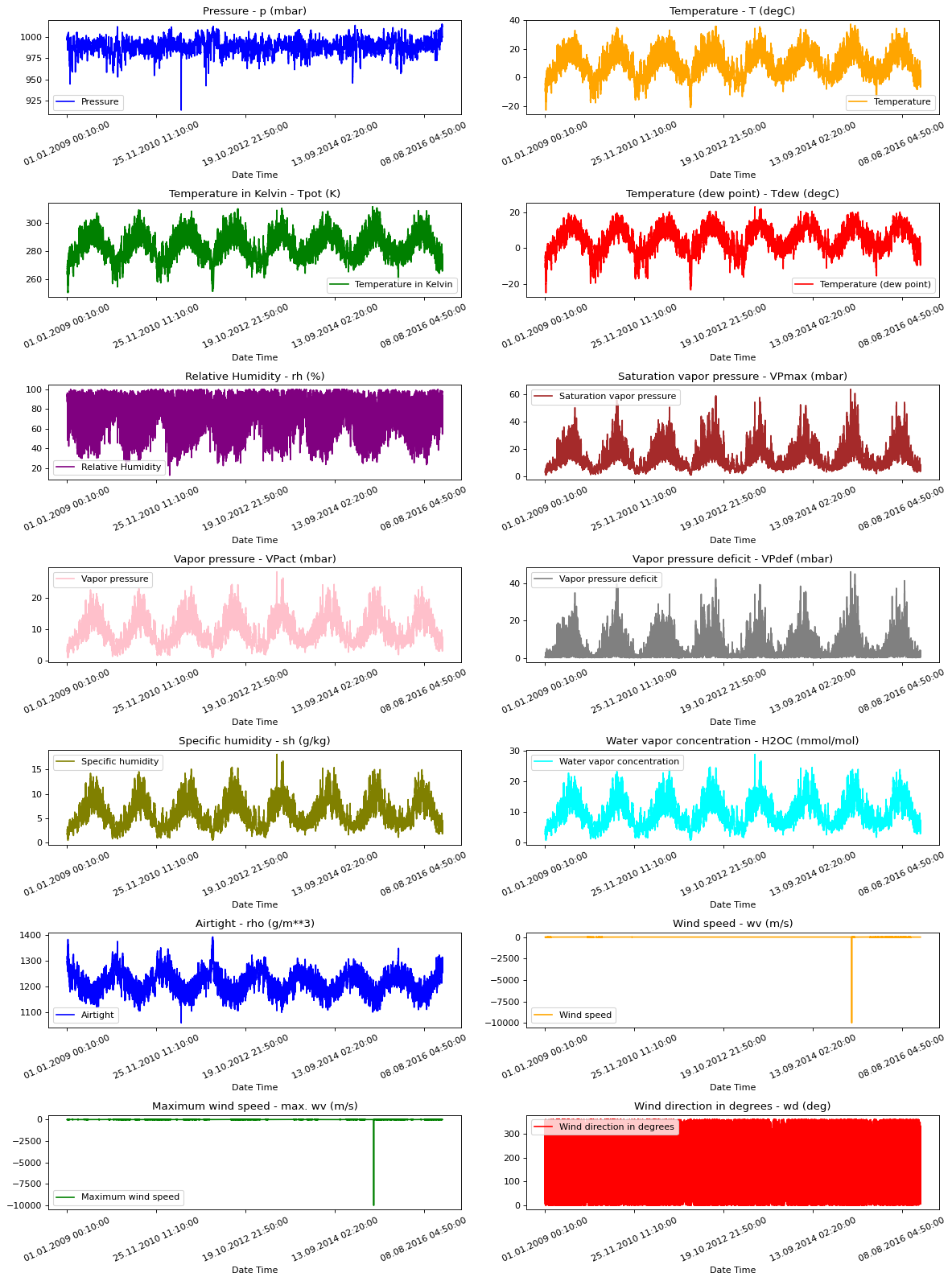This heat map shows the correlation between different features.

``````def show_heatmap(data):
plt.matshow(data.corr())
plt.xticks(range(data.shape), data.columns, fontsize=14, rotation=90)
plt.gca().xaxis.tick_bottom()
plt.yticks(range(data.shape), data.columns, fontsize=14)

cb = plt.colorbar()
cb.ax.tick_params(labelsize=14)
plt.title("Feature Correlation Heatmap", fontsize=14)
plt.show()

show_heatmap(df)
``````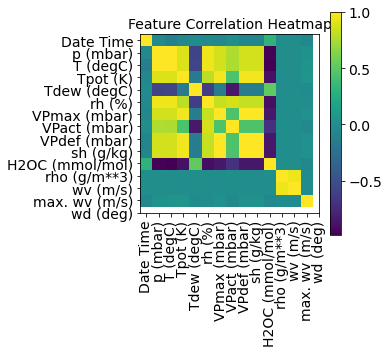## Data Preprocessing

Here we are picking ~300,000 data points for training. Observation is recorded every 10 mins, that means 6 times per hour. We will resample one point per hour since no drastic change is expected within 60 minutes. We do this via the `sampling_rate` argument in `timeseries_dataset_from_array` utility.

We are tracking data from past 720 timestamps (720/6=120 hours). This data will be used to predict the temperature after 72 timestamps (72/6=12 hours).

Since every feature has values with varying ranges, we do normalization to confine feature values to a range of `[0, 1]` before training a neural network. We do this by subtracting the mean and dividing by the standard deviation of each feature.

71.5 % of the data will be used to train the model, i.e. 300,693 rows. `split_fraction` can be changed to alter this percentage.

The model is shown data for first 5 days i.e. 720 observations, that are sampled every hour. The temperature after 72 (12 hours * 6 observation per hour) observation will be used as a label.

``````split_fraction = 0.715
train_split = int(split_fraction * int(df.shape))
step = 6

past = 720
future = 72
learning_rate = 0.001
batch_size = 256
epochs = 10

def normalize(data, train_split):
data_mean = data[:train_split].mean(axis=0)
data_std = data[:train_split].std(axis=0)
return (data - data_mean) / data_std
``````

We can see from the correlation heatmap, few parameters like Relative Humidity and Specific Humidity are redundant. Hence we will be using select features, not all.

``````print(
"The selected parameters are:",
", ".join([titles[i] for i in [0, 1, 5, 7, 8, 10, 11]]),
)
selected_features = [feature_keys[i] for i in [0, 1, 5, 7, 8, 10, 11]]
features = df[selected_features]
features.index = df[date_time_key]

features = normalize(features.values, train_split)
features = pd.DataFrame(features)

train_data = features.loc[0 : train_split - 1]
val_data = features.loc[train_split:]
``````
``````The selected parameters are: Pressure, Temperature, Saturation vapor pressure, Vapor pressure deficit, Specific humidity, Airtight, Wind speed
``````

# Training dataset

The training dataset labels starts from the 792nd observation (720 + 72).

``````start = past + future
end = start + train_split

x_train = train_data[[i for i in range(7)]].values
y_train = features.iloc[start:end][]

sequence_length = int(past / step)
``````

The `timeseries_dataset_from_array` function takes in a sequence of data-points gathered at equal intervals, along with time series parameters such as length of the sequences/windows, spacing between two sequence/windows, etc., to produce batches of sub-timeseries inputs and targets sampled from the main timeseries.

``````dataset_train = keras.preprocessing.timeseries_dataset_from_array(
x_train,
y_train,
sequence_length=sequence_length,
sampling_rate=step,
batch_size=batch_size,
)
``````

## Validation dataset

The validation dataset must not contain the last 792 rows as we won't have label data for those records, hence 792 must be subtracted from the end of the data.

The validation label dataset must start from 792 after train_split, hence we must add past + future (792) to label_start.

``````x_end = len(val_data) - past - future

label_start = train_split + past + future

x_val = val_data.iloc[:x_end][[i for i in range(7)]].values
y_val = features.iloc[label_start:][]

dataset_val = keras.preprocessing.timeseries_dataset_from_array(
x_val,
y_val,
sequence_length=sequence_length,
sampling_rate=step,
batch_size=batch_size,
)

for batch in dataset_train.take(1):
inputs, targets = batch

print("Input shape:", inputs.numpy().shape)
print("Target shape:", targets.numpy().shape)
``````
``````Input shape: (256, 120, 7)
Target shape: (256, 1)
``````

## Training

``````inputs = keras.layers.Input(shape=(inputs.shape, inputs.shape))
lstm_out = keras.layers.LSTM(32)(inputs)
outputs = keras.layers.Dense(1)(lstm_out)

model = keras.Model(inputs=inputs, outputs=outputs)
model.summary()
``````
``````Model: "model"
_________________________________________________________________
Layer (type)                Output Shape              Param #
=================================================================
input_1 (InputLayer)        [(None, 120, 7)]          0

lstm (LSTM)                 (None, 32)                5120

dense (Dense)               (None, 1)                 33

=================================================================
Total params: 5,153
Trainable params: 5,153
Non-trainable params: 0
_________________________________________________________________
``````

We'll use the `ModelCheckpoint` callback to regularly save checkpoints, and the `EarlyStopping` callback to interrupt training when the validation loss is not longer improving.

``````path_checkpoint = "model_checkpoint.h5"
es_callback = keras.callbacks.EarlyStopping(monitor="val_loss", min_delta=0, patience=5)

modelckpt_callback = keras.callbacks.ModelCheckpoint(
monitor="val_loss",
filepath=path_checkpoint,
verbose=1,
save_weights_only=True,
save_best_only=True,
)

history = model.fit(
dataset_train,
epochs=epochs,
validation_data=dataset_val,
callbacks=[es_callback, modelckpt_callback],
)
``````
``````Epoch 1/10
1172/1172 [==============================] - ETA: 0s - loss: 0.2101
Epoch 1: val_loss improved from inf to 0.16601, saving model to model_checkpoint.h5
1172/1172 [==============================] - 89s 75ms/step - loss: 0.2101 - val_loss: 0.1660
Epoch 2/10
1172/1172 [==============================] - ETA: 0s - loss: 0.1288
Epoch 2: val_loss improved from 0.16601 to 0.14444, saving model to model_checkpoint.h5
1172/1172 [==============================] - 87s 75ms/step - loss: 0.1288 - val_loss: 0.1444
Epoch 3/10
1172/1172 [==============================] - ETA: 0s - loss: 0.1147
Epoch 3: val_loss did not improve from 0.14444
1172/1172 [==============================] - 88s 75ms/step - loss: 0.1147 - val_loss: 0.1628
Epoch 4/10
1172/1172 [==============================] - ETA: 0s - loss: 0.1166
Epoch 4: val_loss improved from 0.14444 to 0.13036, saving model to model_checkpoint.h5
1172/1172 [==============================] - 89s 76ms/step - loss: 0.1166 - val_loss: 0.1304
Epoch 5/10
1172/1172 [==============================] - ETA: 0s - loss: 0.1083
Epoch 5: val_loss did not improve from 0.13036
1172/1172 [==============================] - 104s 89ms/step - loss: 0.1083 - val_loss: 0.1392
Epoch 6/10
1172/1172 [==============================] - ETA: 0s - loss: 0.1064
Epoch 6: val_loss did not improve from 0.13036
1172/1172 [==============================] - 131s 112ms/step - loss: 0.1064 - val_loss: 0.1423
Epoch 7/10
1172/1172 [==============================] - ETA: 0s - loss: 0.1041
Epoch 7: val_loss did not improve from 0.13036
1172/1172 [==============================] - 112s 96ms/step - loss: 0.1041 - val_loss: 0.1378
Epoch 8/10
1172/1172 [==============================] - ETA: 0s - loss: 0.1028
Epoch 8: val_loss did not improve from 0.13036
1172/1172 [==============================] - 95s 81ms/step - loss: 0.1028 - val_loss: 0.1369
Epoch 9/10
1172/1172 [==============================] - ETA: 0s - loss: 0.1020
Epoch 9: val_loss did not improve from 0.13036
1172/1172 [==============================] - 84s 72ms/step - loss: 0.1020 - val_loss: 0.1315
``````

We can visualize the loss with the function below. After one point, the loss stops decreasing.

``````def visualize_loss(history, title):
loss = history.history["loss"]
val_loss = history.history["val_loss"]
epochs = range(len(loss))
plt.figure()
plt.plot(epochs, loss, "b", label="Training loss")
plt.plot(epochs, val_loss, "r", label="Validation loss")
plt.title(title)
plt.xlabel("Epochs")
plt.ylabel("Loss")
plt.legend()
plt.show()

visualize_loss(history, "Training and Validation Loss")
``````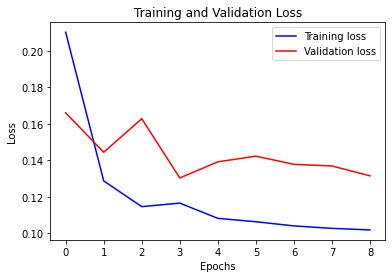## Prediction

The trained model above is now able to make predictions for 5 sets of values from validation set.

``````def show_plot(plot_data, delta, title):
labels = ["History", "True Future", "Model Prediction"]
marker = [".-", "rx", "go"]
time_steps = list(range(-(plot_data.shape), 0))
if delta:
future = delta
else:
future = 0

plt.title(title)
for i, val in enumerate(plot_data):
if i:
plt.plot(future, plot_data[i], marker[i], markersize=10, label=labels[i])
else:
plt.plot(time_steps, plot_data[i].flatten(), marker[i], label=labels[i])
plt.legend()
plt.xlim([time_steps, (future + 5) * 2])
plt.xlabel("Time-Step")
plt.show()
return

for x, y in dataset_val.take(5):
show_plot(
[x[:, 1].numpy(), y.numpy(), model.predict(x)],
12,
"Single Step Prediction",
)
``````
``````8/8 [==============================] - 0s 11ms/step
``````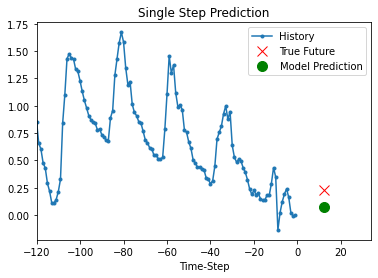``````8/8 [==============================] - 0s 8ms/step
``````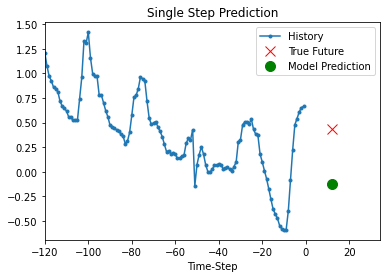``````8/8 [==============================] - 0s 11ms/step
``````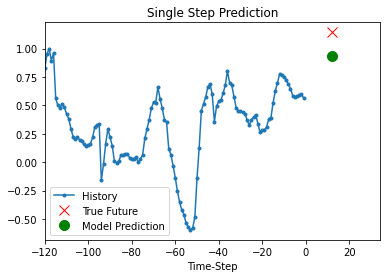``````8/8 [==============================] - 0s 8ms/step
``````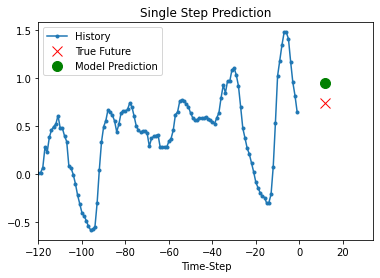``````8/8 [==============================] - 0s 9ms/step
``````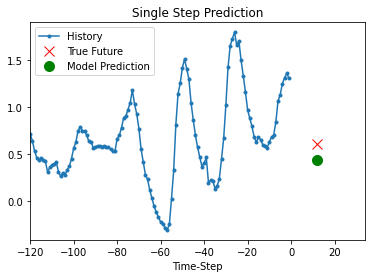Example available on HuggingFace | Trained Model | Demo | | :--: | :--: | |||